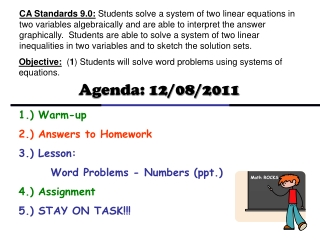DownloadDownload PresentationObjective: ( 1 ) Students will solve word problems using systems of equations.

# Objective: ( 1 ) Students will solve word problems using systems of equations.

Télécharger la présentation## Objective: ( 1 ) Students will solve word problems using systems of equations.

- - - - - - - - - - - - - - - - - - - - - - - - - - - E N D - - - - - - - - - - - - - - - - - - - - - - - - - - -
##### Presentation Transcript

1. CA Standards 9.0: Students solve a system of two linear equations in two variables algebraically and are able to interpret the answer graphically. Students are able to solve a system of two linear inequalities in two variables and to sketch the solution sets. Objective: (1) Students will solve word problems using systems of equations. Agenda: 12/08/2011 1.) Warm-up 2.) Answers to Homework 3.) Lesson: Word Problems - Numbers (ppt.) 4.) Assignment 5.) STAY ON TASK!!! Math ROCKS

2. 8.4 Word Problems - Numbers Notes: Word Problems Type: Numbers “is” means equal Ex 1) The sum of two numbers is 16. Their difference is 8. Find the two numbers. x + y = 16 Letx – Bigger Number y – Smaller Number x – y = 8 + 0 2x = 24 2 2 x = 12 x + y = 16 12 + y = 16 -12 -12 y = 4

3. 8.4 Word Problems - Numbers You try: “is” means equal Ex 2) The sum of two numbers is 35. Their difference is 7. Find the two numbers. x + y = 35 Letx – Bigger Number y – Smaller Number x – y = 7 + 0 2x = 42 2 2 x = 21 x + y = 35 21 + y = 35 -21 -21 y = 14

4. 8.4 Word Problems - Numbers Notes: Word Problems Type: Numbers Ex 3) The sum of two numbers is 25. When four times the smaller number is subtracted from three times the larger number, the result is 5. What is the larger of the two numbers? 4 x + y = 25 4x Letx – Bigger Number = 100 + 4y y – Smaller Number –4y 3x = 5 3x – 4y = 5 7x + 0 = 105 7 7 x = 15 15 is the larger number!!

5. 8.4 Word Problems - Numbers You try: Ex 4) The sum of two numbers is 18. When five times the smaller number is subtracted from six times the larger number, the result is 31. What is the larger of the two numbers? 5 x + y = 18 5x Letx – Bigger Number = 90 + 5y y – Smaller Number –5y 6x = 31 6x – 5y = 31 11x + 0 = 121 11 11 x = 11 11 is the larger number!!

6. 8.4 Word Problems - Numbers Notes: Word Problems Type: Numbers Ex 5) The difference of two numbers is 9. Three times the smaller number plus five times the larger number is 61. What are the two numbers? 3 x – y = 9 3x Letx – Bigger Number = 27 – 3y y – Smaller Number + = 61 3y 5x + 3y = 61 +5x 8x + 0 = 88 8 8 x = 11 x – y = 9 11 – y = 9 -11 -11 – y = -2 y = 2